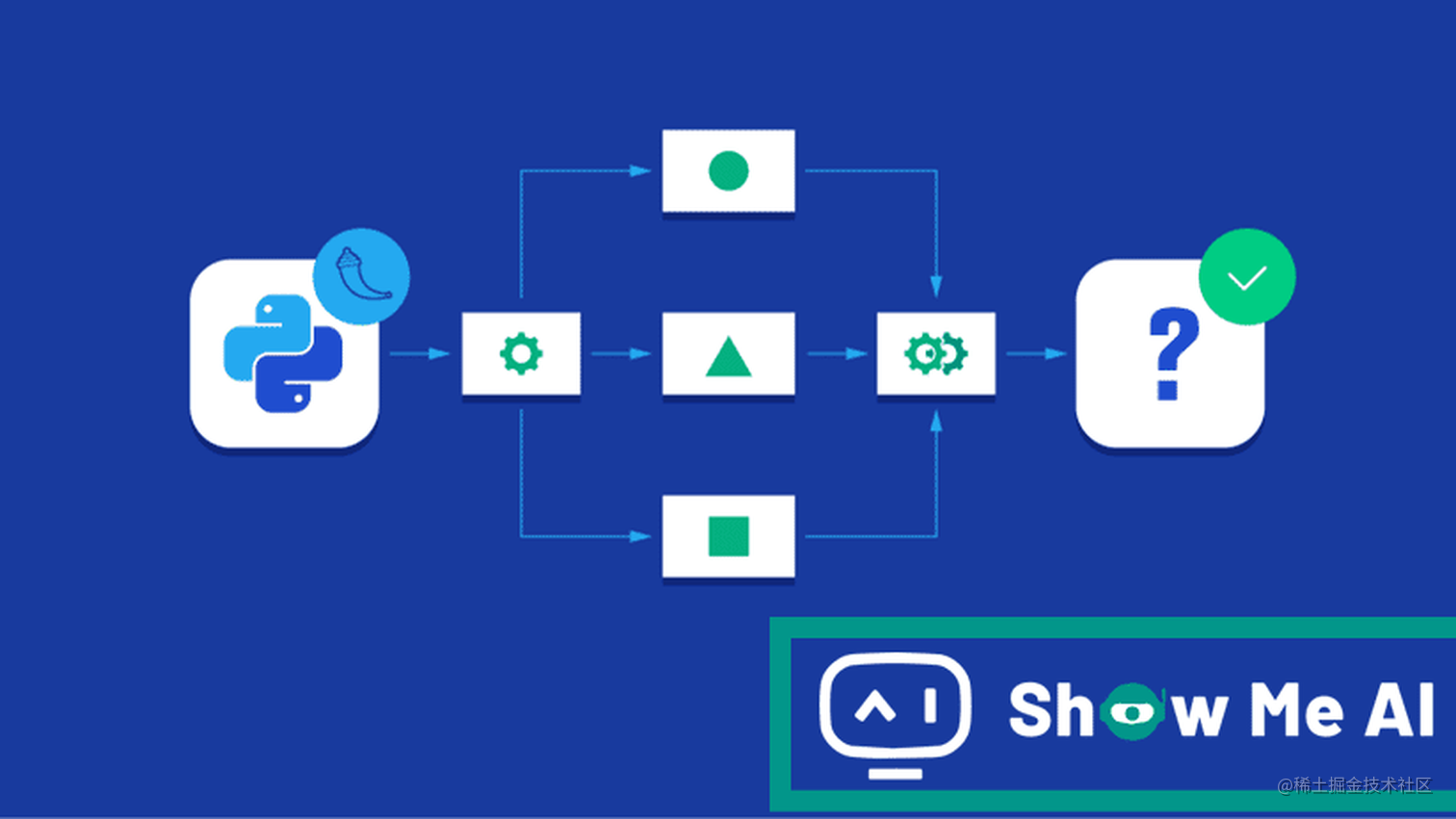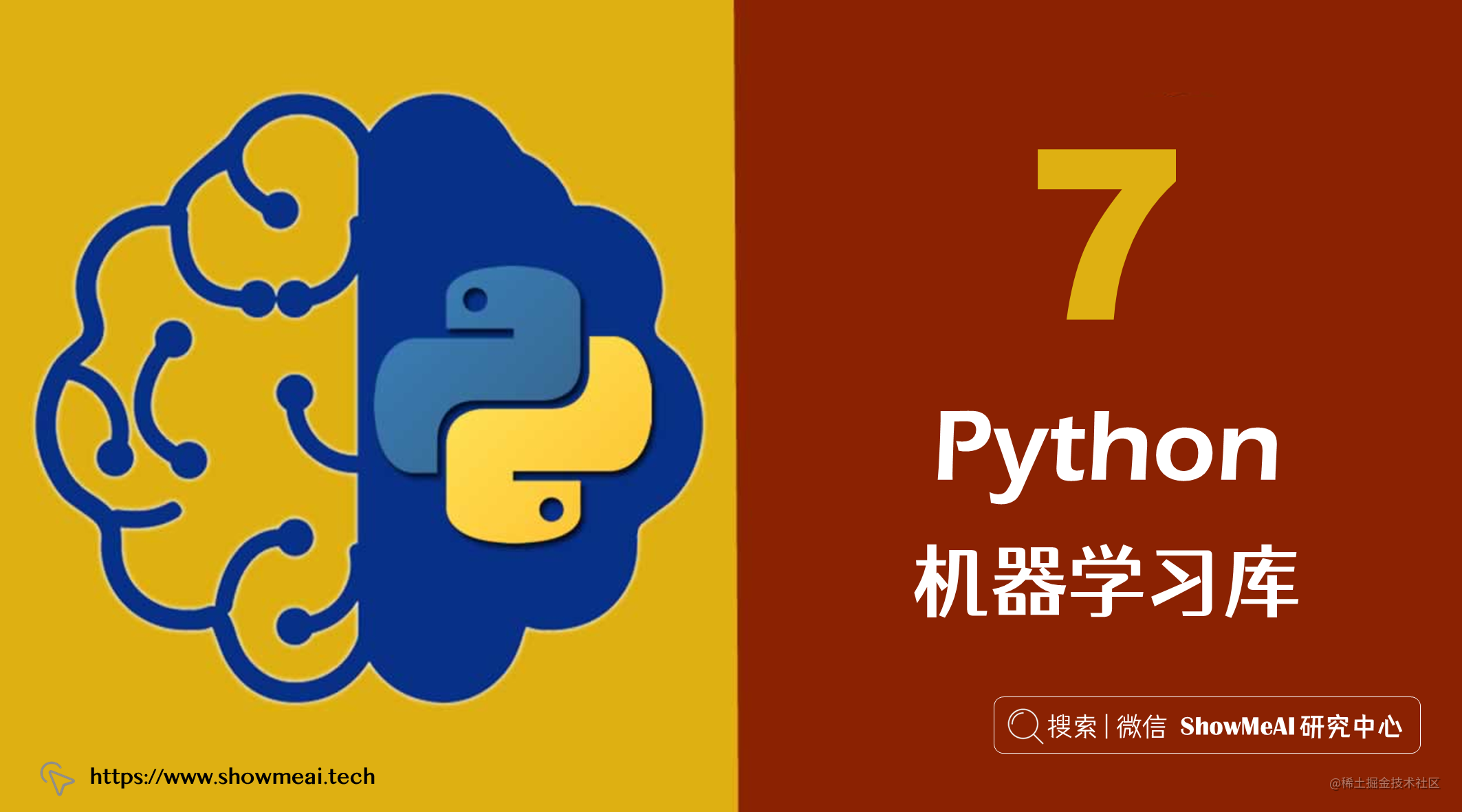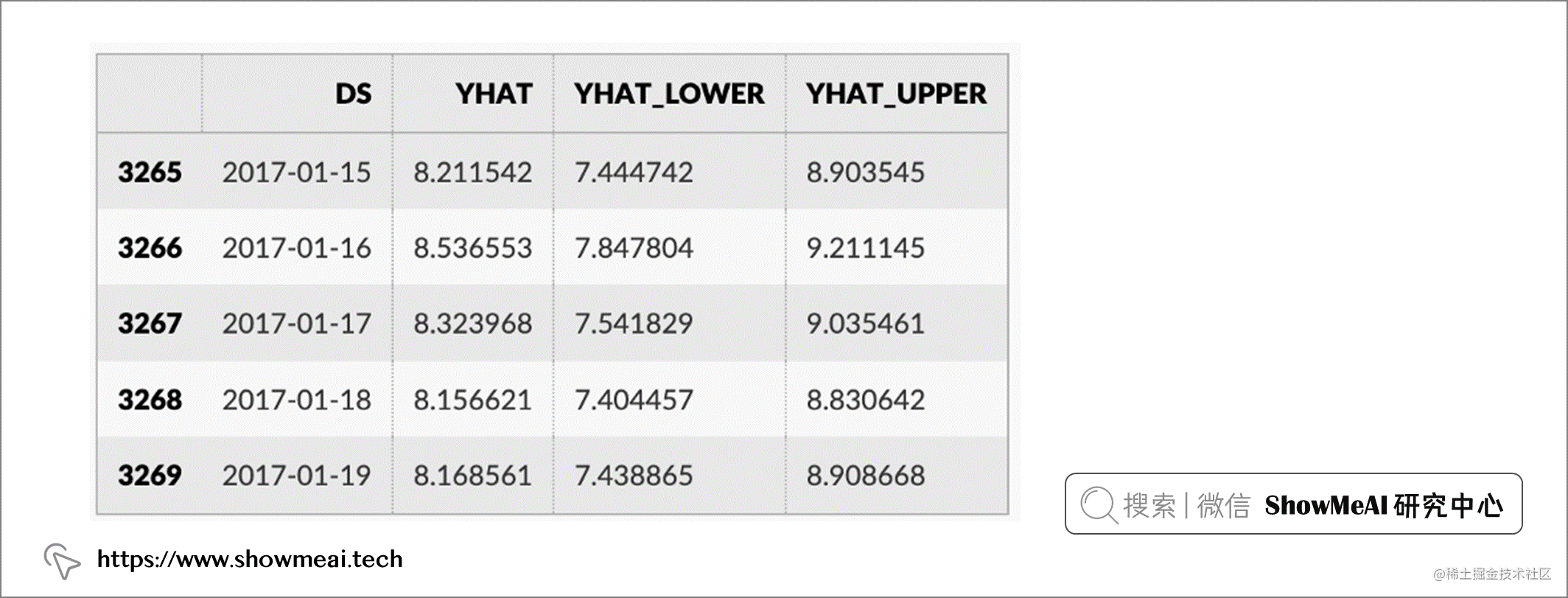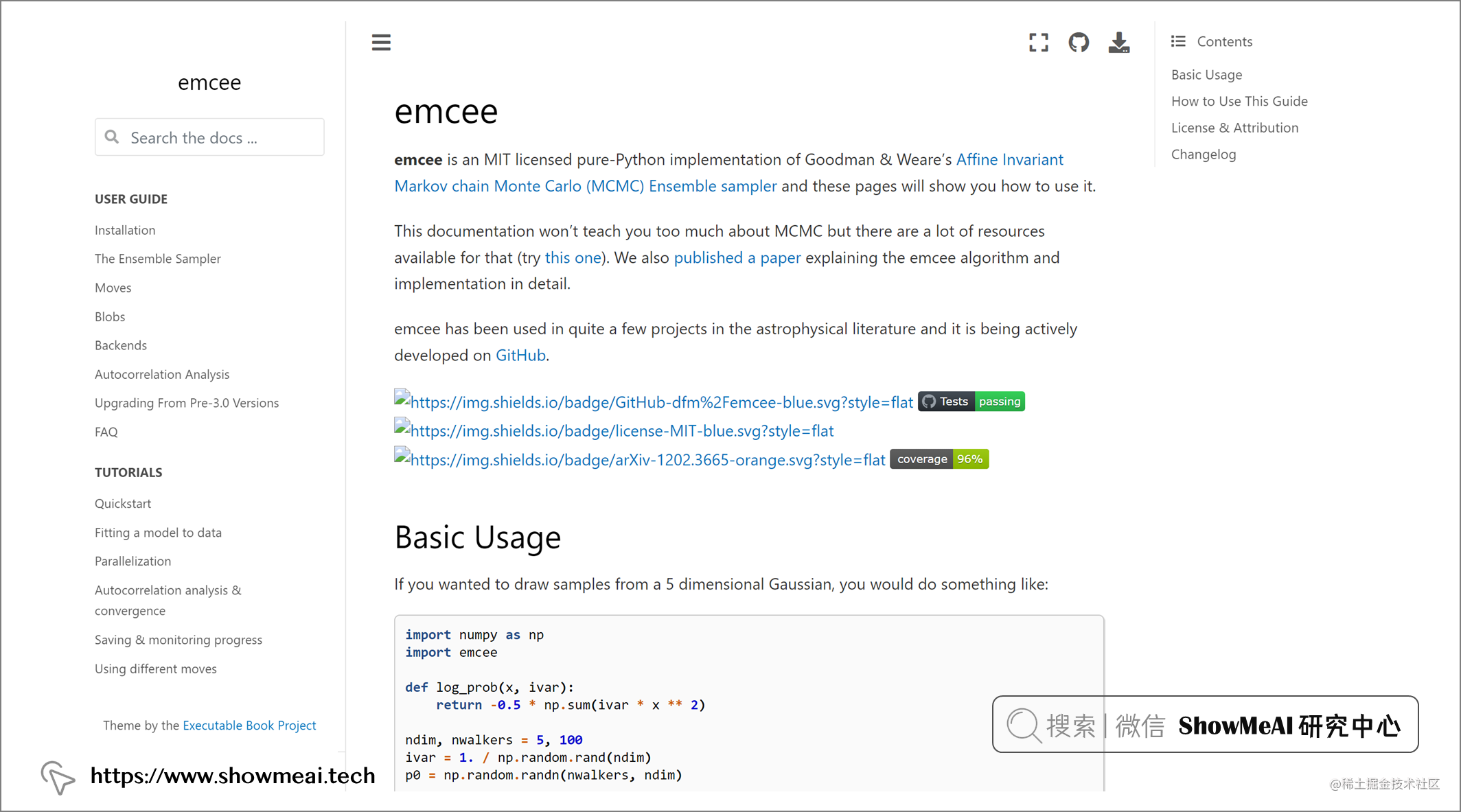# 实用！7个强大的Python机器学习库！⛵# 💡 前言# 💡 工具库介绍

## 💦 1.Prophet

📘Prophet 是 Facebook 开源的时间序列预测工具库，基于 Stan 框架，可以自动检测时间序列中的趋势、周期性和节假日效应，并根据这些信息进行预测。这个库在 GitHub 上有超过 15k 星。Prophet 通常用于预测未来几个月、几年或几十年的时间序列数据，例如销售额、市场份额等。它提供了 Python 和 R 两个版本，可以跨平台使用，支持 CPU 和 GPU 的并行运算。Prophet 的输入数据格式要求是一个包含时间戳和目标值的数据框，并支持给定时间范围、预测期限和宽限期等参数进行预测。Prophet 对缺失数据和趋势变化很稳健，通常可以很好地处理异常值。

``````# Python
forecast = m.predict(future)
forecast[['ds', 'yhat', 'yhat_lower', 'yhat_upper']].tail()## 💦 2.Deep Lake

📘Deep Lake 是一种数据集格式，提供简单的 API 以用于创建、存储和协作处理任何规模的 AI 数据集。这个库在 GitHub 上有超过 5k 星。Deep Lake 的数据布局可以在大规模训练模型的同时，实现数据的快速转换和流式传输。谷歌、Waymo、红十字会、牛津大学等都在使用 Deep Lake。

``````for epoch in range(2):
running_loss = 0.0
images, labels = data['images'], data['labels']

# forward + backward + optimize
outputs = net(images)
loss = criterion(outputs, labels.reshape(-1))
loss.backward()
optimizer.step()

# print statistics
running_loss += loss.item()
if i % 100 == 99: #print every 100 mini-batches
print('[%d, %5d] loss: %.3f' %
(epoch + 1, i + 1, running_loss / 100))
running_loss = 0.0

## 💦 3.Optuna

📘Optuna 是一个自动机器学习超参数调优工具，可以帮助用户通过使用各种规则自动调整机器学习模型的超参数，以提高模型的性能。这个库在 GitHub 上拥有超过 7k 颗星。Optuna 使用了贝叶斯优化算法来自动调整超参数，并使用基于树的方法来探索参数空间。这使得 Optuna 能够在训练机器学习模型时自动进行超参数调整，从而提高模型的性能。Optuna 可以与各种机器学习框架集成使用，包括 TensorFlow、PyTorch、XGBoost 等。它还支持多种优化目标，包括最小化损失函数、最大化准确率等。

``````import ...

# Define an objective function to be minimized.
def objective(trial):

# Invoke suggest methods of a Trial object to generate hyperparameters

regressor_name = trial.suggest_categorical('regressor',['SVR', 'RandomForest'])
if regressor_name = 'SVR':
svr_c = trial.suggest_float('svr_c', 1e-10, 1e10, log=True)
regressor_obj = sklearn.svm.SVR(C=svr_c)
else:
rf_max_depth = trial.suggest_int('rf_max_depth', 2, 332)
regressor_obj = sklearn.ensemble.RandomForestRegressor(max_depth=rf_max_depth)

X, y = sklearn.datasets.fetch_california_housing(return_X_y=True)
X_train, X_val, y_train, y_val = sklearn.model_selection.train_test_split(X, y, random_state=0)

regressor_obj.fit(X_train, y_train) y_pred = regressor_obj.predict(X_val)

error = sklearn.metrics.mean_squared_error(y_val, y_pred)

return error # An objective value linked with the Trial object.

study = optuna.create_study() # Create a neW study
study.optimize(objective, n_trials=100) # Invoke opotimization of the objective function

## 💦 4.pycm

📘pycm 是一个用于计算二分类和多分类指标的 Python 库。这个库在 GitHub 上有超过 1k 星。``````from pycm import *
y_actu = [2, 0, 2, 2, 0, 1, 1, 2, 2, 0, 1, 2]
y_pred = [0, 0, 2, 1, 0, 2, 1, 0, 2, 2, 2, 2]

cm = ConfusionMatrix(actual_vector=y_actu, predict_vector=y_pred)

cm.classes

cm.print_matrix()

cm.print_normalized_matrix()

## 💦 5.NannyML

📘NannyML 是一个开源的 Python 库，允许估算部署后的模型性能（而无需访问目标），检测数据漂移，并智能地将数据漂移警报链接回模型性能的变化。这个库在 GitHub 上有超过 1k 星。``````import nannyml as nml
from IPython.display import display

# Choose a chunker or set a chunk size
chunk size = 5000

# initialize, specify required data columns,, fit estimator and estimate
estimator = nml.CBPE(
y_pred_proba='y_pred_proba',
y_pred='y_pred',
y_true='work_home_actual',
metrics=['roc_auc'],
chunk_size=chunk_size,
problem_type='classification_binary',
)
estimator = estimator.fit(reference)
estimated_performance = estimator.estimate(analysis)

# Show results
figure = estimated_performance.plot(kind='performance', metric='roc_auc', plot_reference=True)
figure.show()

## 💦 6.ColossalAI

📘ColossalAI 是一个开源机器学习工具库，用于构建和部署高质量的深度学习模型。这个库在 GitHub 上有超过 6.5k 星。ColossalAI 提供了一系列预定义的模型和模型基础架构，可用于快速构建和训练模型。它还提供了一系列工具，用于模型评估，调优和可视化，以确保模型的高质量和准确性。此外，ColossalAI 还支持部署模型，使其能够通过各种不同的接口与其他系统集成。ColossalAI 的优势在于它易于使用，可以为数据科学家和机器学习工程师提供快速和有效的方法来构建和部署高质量的大型模型。

``````from colossalai.logging import get_dist_logger
from colossalai.trainer import Trainer, hooks

# build components and initialize with colossaalai.initialize
...

# create a logger so that trainer can log on thhe console
logger = get_dist_logger()

# create a trainer object
trainer = Trainer(
engine=engine,
logger=logger
)

## 💦 7.emcee

📘emcee 是一个开源的 Python 库，用于使用 Markov chain Monte Carlo（MCMC）方法进行模型拟合和参数估计。这个库在 GitHub 上有超过 1k 星。emcee是面向对象的，并且具有用于诊断和调试拟合过程的许多工具。它使用了一种叫做"决策树结构链"的方法，可以并行化拟合过程，提高拟合效率。emcee 非常适合处理复杂的非线性模型，并且可以轻松扩展到大型数据集。它也可以轻松与其他 Python 库集成，如 NumPy、SciPy和Matplotlib。

``````import numpy as np
import emcee

def log_prob(x, ivar):
return -0.5 * np.sum(ivar * x ** 2)

ndim, nwalkers = 5, 100

ivar = 1./np.random.rand(ndim)
p0 = np.random.randn(nwalkers, ndim)

sampler = emcee.EnsembleSampler(nwalkers, ndim, log_prob, args=[ivar])
sampler.run_mcmc(p0, 10000)

# 推荐阅读